Journal homepage ISSN: 1303 - 2968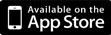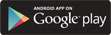©Journal of Sports Science and Medicine (2010) 09, 86 - 91 Research article Four Ball Best Ball 1 Geoff Pollard1, Graham Pollard2,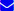Author Information 1 Faculty of Life and Social Sciences, Swinburne University of Technology, Melbourne, Australia 2 Faculty of Information Sciences and Engineering, University of Canberra, Australia Graham Pollard✉ Faculty of Information Sciences and Engineering, University of Canberra, ACT 2601, AustraliaEmail: graham@foulsham.com.au Publish Date Received: 18-09-2009 Accepted: 13-12-2010 Published (online): 01-03-2010 × Geoff Pollard, Graham Pollard. (2010) Four Ball Best Ball 1. Journal of Sports Science and Medicine (09), 86 - 91.  Email link to this article × Geoff Pollard, Graham Pollard. (2010) Four Ball Best Ball 1. Journal of Sports Science and Medicine (09), 86 - 91. Your name: Your E-mail: Recipient’s E-mail:
 Abstract Introduction Methods Results Discussion Author biography References

ABSTRACT

In this paper a four-ball-best-ball (4BBB) model for pairs of golf players is set up. The 4BBB match-play scoring system is seen to satisfy a basic requirement of fairness. It is shown that it is not strictly possible to rate individual players as 4BBB players. However, a (reasonably broad) class of individual players is identified such that it is possible to rate them individually as 4BBB players. The capacity of an individual to play birdies is seen to be a very important determinant in being a successful member of a 4BBB pair, but there are other minor factors as well. Consideration is given to equal and unequal 4BBB pairs. The transitive law is seen to apply for 4BBB pairs. Thus, if pair A is better than pair B, and pair B is better than pair C, then pair A must be better than pair C. Correspondingly, if pair A is equal to pair B, and pair B is equal to pair C, then pair A is equal to pair C. Consideration is given to some strategic issues in 4BBB match-play golf. For example, the conditions under which a player should take a greater risk and have a higher probability of obtaining a bogie in order to achieve a higher probability of scoring a birdie, are determined. Also, the conditions under which a player, noting that his partner is about to have a ‘bad’ hole and score only a par or a bogie, should ‘play safe’, are determined. Thirdly, players who can interact in certain ways are seen to have an advantage over those pairs that cannot do this. Finally, one pair’s optimal strategy when they see that their opponents are about to score a par or a bogie, but not a birdie, is analyzed.

Key words: Strategies for four ball best ball golf, selecting pairs of four ball best ball players

Key Points
• A model for four-ball-best-ball match-play golf is established, and used to show that, although there can be other factors, the capacity of an individual to play birdies is a very important determinant in that player being a successful member of a four-ball-best-ball pair.
• Although it is not possible in general to rate play-ers individually as 4BBB players, a class of indi-vidual players is identified such that rating the players within that class is possible.
• Equal and unequal 4BBB pairs are considered, and the transitive law is seen to apply for 4BBB pairs. For example, if pair A is better than pair B, and pair B is better than pair C, then pair A must be better than pair C.
• Several strategic issues for individual players and pairs of players are considered, and optimal strate-gies identified.

 INTRODUCTION

In four-ball-best-ball match-play golf, two players (A and B) play as a pair against two other players (C and D) who also play as a pair. All players play in the usual way. On the first hole, pair (A, B)’s score is the minimum number of strokes A or B took to complete the hole. Correspondingly, pair (C, D)’s score on the first hole is the minimum number of strokes C or D took to complete the hole. One pair wins the hole if their minimum score is less than the other pair’s minimum score, and 1 is added to their match score. The match ceases as soon as one pair has an unbeatable lead and/or 18 holes have been completed. If the match is tied or ‘squared’ after the 18th hole has been played, more holes may be played until a winner is determined by a lead by 1. This is called a ‘playoff’.

In this paper several questions are addressed. What is a useful model for studying 4BBB golf? Is the scoring system fair? Can individual players be ranked even though they are playing as pairs? Can pairs of players be ranked or can cyclical relationships (pair A better than pair B, who is better than pair C, who is better than pair A) exist between several pairs? Is it possible for pair A to be stronger than pair B at 4BBB golf, but weaker at other forms of the game? Are birdies particularly important in winning at 4BBB golf? Can two players make strategic decisions to interact in a useful way on a particular hole, and on any hole in general? Can such decisions be usefully made both statically and dynamically?

In a recent paper, Hurley (2007) studied how a given set of players might be combined into pairs so as to form a very effective team against an identical opposing team. This is studied in a second paper by the authors (Pollard and Pollard, 2008).

 METHODS

The four-ball-best-ball model

For PGA players playing 4BBB match-play, a pair’s score on a hole is typically a birdie (-1), a par (0) or a bogie (+1). For top amateurs and A-grade players, quite often playing on easier courses, the same is true. Even for average players, typically playing on easier courses, this is also true. Thus, a useful model for 4BBB match play golf is to denote pair A by {a-1, a0, a+1} where a-1 is the probability pair A gets a birdie, a0 is the probability pair A gets a par, and a+1 is the probability they get a bogie on any hole. Correspondingly, pair B can be denoted by {b-1, b0, b+1}. If we assume that pair A’s score and pair B’s score on any hole are independent, it can be seen that the probability pair A wins a hole against pair B, pA, is given by a-1(1 - b-1) + a0b+1, and the probability pair B wins a hole against pair A is given by pB = b-1(1 - a-1) + b0a+1. The probability that the hole is squared is given by 1 - pA - pB. The probability that pair A wins (loses) an 18-hole 4BBB match-play event, PA (PB), can be evaluated using recurrence methods, and the probability that the match is squared is equal to 1 - PA - PB. If a play-off is used when the 18 holes are squared, the probability pair A wins (loses) the match can be evaluated using the geometric distribution.

A further aspect of the model

We suppose players A1 and A2 obtain scores for pair A (birdie, par or bogie) that are independently distributed, and we denote player A1 by (pa1,-1, pa1,0, pa1,+1) and player A2 by (pa2,-1, pa2,0, pa2,+1). The pair A, playing independently, is denoted by {(pa1,-1, pa1,0, pa1,+1), (pa2,-1, pa2,0, pa2,+1)}. A corresponding notation is used for pair B, and it is typically assumed that pair A’s score on a hole is independent of pair B’s score on the hole. For a hole for team A, a-1 = pa1,-1 + pa2,-1 - pa1,-1pa2,-1, a+1 = pa1,+1pa2,+1 and a0 = 1 - a-1 - a+1, and corresponding expressions apply for pair B.

‘Fairness’ of the four-ball-best-ball match-play scoring system

A minimal criterion for fairness of the 4BBB scoring system would appear to be the requirement that if pairs A and B are identical, the ‘hole-probabilities’ against each other pA and pB must be equal, and hence PA must equal PB. It is clear that this requirement is satisfied, since if a-1 is equal to b-1, a0 is equal to b0 and a+1 is equal to b+1, pA must equal pB and PA must equal PB. For the basic model above, it is clear that if player A1 is identical to player B1, and player A2 identical to B2, we must have pA = pB, and PA must equal PB.

Example 1. Noting that PGA tour players score a birdie approximately 20% of the time on average, we consider in this example four identical players, each with a probability of 0.2 of scoring a birdie, a probability 0.6 of scoring a par, and a probability 0.2 of scoring a bogie. It would seem that these probabilities would also be applicable to top amateurs and top club players on somewhat easier courses. The probability pair A wins a hole against pair B is equal to pA = 0.2544, the probability pair B wins a hole against pair A is equal to pB = 0.2544, and the probability that the hole is halved is equal to 0.4912. Recurrence methods can be used to show that the probability pair A wins an 18-hole match is equal to 0.4346, the probability pair B wins it is also 0.4346, whilst the probability that the 18-holes are squared is equal to 0.1309. After a play-off if necessary, the probability each pair wins is equal to 0.5. These results are shown in Table 1, and this example demonstrates the ‘fairness’ of the 4BBB scoring system.

Example 2. In this example the pair A has characteristics {(0.25, 0.5, 0.25), (0.25, 0.5, 0.25)} and the pair B has characteristics {(0.2, 0.6, 0.2), (0.2, 0.6, 0.2)}. It can be seen from table 1 that pair A now has a probability of 0.6417 of winning an 18-hole match with playoff against pair B. Pair A’s increased probability of winning the match (compared with their value in example 1) is essentially due to the role of a-1 in the expression pA = a-1(1 - b-1) + a0b+1. Note that it can easily be argued that all four players in this example are equal golfers, as their expected scores on every hole are the same (and equal to 0). Pair A is, however, the better 4BBB pair. The 4BBB format of scoring has favoured the more variable pair, pair A. Note further that if we increased pair A’s probability of a bogie (or decreased their probability of a birdie) very slightly and decreased (increased) their probability of a par accordingly, so that pair A’s expected score was slightly less than that of pair B, we would still have a situation in which pair A was the better 4BBB pair. This might seem incongruous to believers in stroke play and other golf formats. Thus, it is clearly possible to have a pair that is better than another pair at 4BBB golf, whilst being weaker at other forms of the game.

Rating individual four-ball-best-ball players

In this section we investigate whether it is possible to rank individual players as 4BBB players.

Example 3. Suppose y = (p, 1 - 2p, p) and x = (p - ɛ, 1 - p + ɛ, 0) are two types of players where 0 < ɛ < p < 0.5. Consider a 4BBB match between pair A with characteristics {x, y} and pair B with characteristics {x, x}. It can be shown that pair A is always the better pair (better since pA > pB), and this therefore suggests that y is a better 4BBB player than x. However, when we consider a 4BBB match between pair A with characteristics {y, x} and pair B with characteristics {y, y}, we find that pair A is the better 4BBB pair provided ɛ is sufficiently small so as to satisfy the equation p4 - (2 + ɛ)p3 + (ɛ + 1)p2 + ɛp - ɛ > 0. For example, when p = 0.2, ɛ needs to be less than 1/30. Thus, provided ɛ is sufficiently small, this result suggests that x is a better 4BBB player than y. Therefore, we have, for small ɛ, one result suggesting that x is a better 4BBB player than y, and another result suggesting that y is a better 4BBB player than x. The truth is that, for small ɛ, x combines more successfully with y than does either x with x, or y with y. These two examples with p = 0.2 and ɛ = 0.01 appear in table 1 as examples 3a and 3b respectively.

As another example, suppose w = (p + ɛ, 1 - 2p - 2ɛ, p + ɛ), x = (p + ɛ, 1 - p - ɛ, 0), y = (p, 1 - 2p, p) and z = (p - ɛ, 1 - p + ɛ, 0) are four types of players where 0 < ɛ < p < 0.5. Consider a 4BBB match between pair A with characteristics {w, x} and pair B with characteristics {z, x}. It can be shown that pair A is always the better pair, suggesting that w is a better 4BBB player than z. However, when we consider a 4BBB match between pair A with characteristics {z, y} and pair B with characteristics {w, y}, we find that pair A is the better 4BBB pair provided ɛ is sufficiently small. (For example, when p = 0.2, provided ɛ is less than about 0.017 (to 0.018)). Thus, provided ɛ is sufficiently small, this second result suggests that z is a better 4BBB player than w. So, again we have a contradiction when ɛ is small. For the case in which p = 0.2 and ɛ = 0.01, see examples 3c and 3d in table 1.

Thus, it can be concluded that it is not possible to strictly/generally rank individual 4BBB players in an order from best to worst. We will see however that it is possible to rank 4BBB pairs. Thus, it is clear that the sum of the birdie probabilities of the players in a pair of 4BBB players tells us a great deal about the strength of that pair, but it is not the complete picture. The following example highlights this point.

Example 4. We start by assuming players B1 and B2 are the same as in example 1, that pair A’s characteristics are {(0.3, 0.5, 0.2), (0.1, 0.7, 0.2)}, rather than {(0.2, 0.6, 0.2), (0.2, 0.6, 0.2)}. Note that the sum of pair A’s birdie probabilities are the same as in example 1, as are the sum of pair A’s par probabilities, and the sum of pair A’s bogie probabilities. However, the interaction between player A1’s and A2’s birdie outcomes is now smaller than before (0.03 instead of 0.04). This reduces the likelihood of players A1 and A2 having birdies at the same time, and this increases their probability of winning an 18-hole match (with playoff) from 0.5 to 0.5238, as can be seen in example 4a of table 1. If pair A’s characteristics change to {(0.3, 0.6, 0.1), (0.1, 0.6, 0.3)}, their probability of winning an 18-hole match against pair B (with playoff) increases further to 0.5398. Note that the probability that both A1 and A2 have a bogie has decreased by 0.01 (from 0.04 to 0.03), as can be seen in example 4b of table 1. If pair A’s characteristics are changed to {(0.3, 0.6, 0.1), (0.1, 0.7, 0.2)}, their probability of winning an 18-hole match against pair B (with playoff) increases further to 0.5560. The probability that both A1 and A2 have a bogie has decreased by 0.02 (from 0.04 to 0.02), as can be seen in example 4d in table 1. If pair A’s characteristics are changed to {(0.3, 0.6, 0.1), (0.1, 0.8, 0.1)}, their probability of winning an 18-hole match (with playoff) increases further to 0.5724, as their probability of both having a bogie has decreased by a further amount 0.01 (from 0.02 to 0.01), as can be seen in example 4e of table 1. Finally, if pair A’s characteristics are changed to {(0.3, 0.6, 0.1), (0.1, 0.9, 0.0)}, their probability of winning an 18-hole match (with playoff) increases further to 0.5888, as can be seen in example 4f of table 1.

Example 5. In this example we see that if the ‘gap in the performance characteristics’ between player A and player B is ‘sufficiently large’, player A can be a better 4BBB player than player B no matter what are the common characteristics of player A’s and player B’s partners. Supposing the two partners with common characteristics are denoted by X, pair {A, X1} can be represented by {(pa,-1, pa,0, pa,-1), (x-1, x0, x+1)}, and pair {B, X2} can be represented by {(pb,-1, pb,0, pb,-1), (x-1, x0, x+1)}. The probability pair {A, X1} wins a hole against pair {B, X2}, pA, can be shown to equal

A corresponding expression can be written down for pB, the probability pair {B, X2} wins a hole against pair {A, X1}. It can be shown that pA > pB provided

Noting that x+1 [0, 1], it can be seen, for example, that player (0.3, 0.6, 0.1) is always a better 4BBB player than player (0.1, 0.8. 0.1), and that player (0.3, 0.4, 0.3) is a better 4BBB player than player (0.1, 0.9, 0.0) provided x+1 for the players with the common characteristics is less than 20/27.

Example 6a. As special case of example 5, we consider the important family of ‘symmetric’ players (p, 1-2p, p). Suppose player A has p = p1 and player B has p = p2, where p1 > p2 > 0. Then it can be seen that player A is always a better 4BBB player than player B (unless x+1 = 1, which is the (irrelevant) case in which the partners with the common characteristics always score bogies).

Example 6b. As another special case of example 5, we consider the family of ‘equally non-symmetric’ players (θp, 1 - (1 + θ)p, p), where θ ≠ 1 and 0 < θ < p-1 - 1. Supposing θ is fixed, player A has p = p1 and player B has p = p2, where p1 > p2 > 0, it can be shown that player A is always a better 4BBB player than B, provided x1 < θ and x-1 ≠ 1. Further, for all (fixed) θ such that 0 < θ < p-1 - 1 (i.e. the symmetric (θ = 1) as well as the non-symmetric (θ ≠ 1)cases), if players C and D have p-values equal to p3 and p4 where p1 > p2 > p3 > p4 > 0, and p1 + p4 = p2 + p3, it can be shown that the pair (A, D) is always a better 4BBB pair than (B, C).

Equal and unequal pairs

Definition. Two independent 4BBB pairs A and B (i.e. two 4BBB pairs A and B whose scores on a hole are independent) are said to be equal 4BBB pairs if pA = pB.

Theorem. If independent pairs A and pair B are equal 4BBB pairs, and if independent pairs B and pair C are also equal 4BBB pairs, it follows that independent pairs A and C are also equal 4BBB pairs.

Proof. Since pair A is equal to pair B,

a′1(1 ′ b′1) + a0b+1 = b1(1 ′ a′1) + b0a+1 , and substituting a0 = 1 ′ a′1a+1 and b0 = 1 ′ b′1b+1 , and subtracting 1 from each side of the equation, we obtain a′1 + b+1a′1b+1 ′ 1 = b′1 + a+1a+1b′1 ′ 1, and hence (a′1 ′ 1)(1 ′ b+1) = (b′1 ′ 1)(1 ′ a+1) .

Correspondingly, since pair B is equal to pair C, we have (b′1 ′ 1)(1 ′ c+1) = (c′1 ′ 1)(1 ′ b+1), and (a′1 ′ 1)(1 ′ c+1) = ((b′1 ′ 1)(1 ′ a+1) / (1 ′ b+1)) hence ((c′1 ′ 1)(1 ′ b+1) / (b′1 ′ 1)) since 1 ′ b+1 and b′1 ′ 1 are not equal to zero, and hence (a′1 ′ 1)(1 ′ c+1) = (c′1 ′ 1)(1 ′ a+1), and hence a′1(1 ′ c′1) + a0c+1 = c′1(1 ′ a′1) + c0a+1 , and so pair A and pair C are equal, completing the proof.

Example 7. If independent pairs A and B are {(p1, 1-2p1, p1), (p2, 1-2p2, p2)} and {(p1, 1-p1-p2, p2), (p2, 1-p1-p2, p1)} respectively, it can be seen that a-1 = b-1, a+1 = b+1 and hence a0 = b0, and it follows that the pairs A and B are equal for all legitimate values of p1 and p2.

Example 8. The pair-wise independent pairs A, B and C given by {(0.25, 0.5, 0.25), (0.25, 0.5, 0.25)}, {(0.25, 0.5, 0.25), (0.3, 0.2, 0.5)} and {(0.25, 0.5, 0.25), (0.2, 0.8, 0)} can be seen to be pair-wise equal. Similarly, the pair-wise independent pairs D, E and F given by {(0.2, 0.6, 0.2), (0.2, 0.6, 0.2)}, {(0.2, 0.6, 0.2), (7/30, 11/30, 0.4)} and {(0.2, 0.6, 0.2), (1/6, 5/6, 0)} also can be seen to be equal.

We have seen above that it is not strictly possible to rank individual 4BBB players. However, it is possible to rank pairs of players as a result of the following theorem.

Definition. Given two independent 4BBB pairs A and B, pair A is said to be the better pair if pA > pB.

Theorem. (transitive theorem) Given three pair-wise independent pairs A, B and C, with A better than B, and B better than C, it follows that A is better than C.

Proof. This theorem can be proved in an analogous manner to the theorem above.

Example 9. Here we reconsider example 7 with p1 = p + ∂ and p2 = p - ∂. Then, given 0.5 > p > ∂2 > ∂1 > 0, it follows that pairs A and B with ∂ = ∂2 are better 4BBB pairs than pairs A and B with ∂ = ∂1.

Example 10. Here we return to example 8 and consider the pair-wise independent pairs G, H and J given by {(0.15, 0.7, 0.15), (0.15, 0.7, 0.15)}, {(0.15, 0.7, 0.15), (39/230, 61/115, 0.3)} and {(0.15, 0.7, 0.15), (3/23, 20/23, 0)} respectively. It can be verified that these three pairs are equal pairs. Then, assuming the nine pairs A, B, C, D, E, F, G, H and J are pair-wise independent, it can be seen that any of A, B and C, are better than any of D, E and F, who in turn are all better than any of G, H and J. Note however, for example, that although A, B and C are equal pairs, and D, E and F are also equal pairs, pA, pB and pC take on 9 different values when playing D, E or F, as can be seen in Table 2.

Some strategy considerations for players and pairs

Example 11. Suppose pair A has characteristics {(p1 + ɛ, 1 - p1 - p2 - ɛ - ∂, p2 + ∂), (p1, 1 - p1 - p2, p2)} and pair B has characteristics {(p1, 1 - p1 - p2, p2), (p1, 1 - p1 - p2, p2)}. In this example players A2, B1 and B2 are identical players. Player A1 is very similar to these three players except that he can, if he wishes, increase his probability of getting a birdie by ɛ > 0 by taking a strategic risk, but in the process his probability of getting a bogie increases by ∂ > 0. It can be shown that pair A’s probability of winning a hole is greater than pair B’s provided ∂ < ((1 - p22)/p2(1 - p1)) ɛ. For example when p1 = p2 = 0.2, this inequality is ∂ < 6ɛ, indicating that for these values of p1 and p2, player A1 can suffer a considerable increase in his bogie probability and it still is worthwhile taking the risk.

Example 12. Assume pairs A and B both have characteristics {(p1, 1 - p1 - p2, p2), (p1, 1 - p1 - p2, p2)}. However, we make an additional assumption concerning player A1. It is assumed that player A1 can detect on a particular hole when his partner, A2, cannot possibly get a birdie, but will get a bogie with probability z and a par with probability 1-z. (For example, if player A2 always tees off first on every hole, such an event can sometimes be immediately clear to player A1 if player A2 plays a very bad first shot). We assume player A1 can take evasive action and reduce his own probability of a bogie by x, but in doing so he reduces his probability of scoring a birdie by an amount y. The question is whether player A1 should, in this situation, take this evasive action. Let us suppose that he will take this evasive action if he can reduce pair B’s probability of winning the hole. If he does not take the evasive action, pair B’s probability of winning the hole is equal to pB = (2p1 - p12)(1-p1) + ((1-p1)2 - p22)zp2 , whereas if he does take the evasive action pB = (2p1 - p12)(1-p1 + y)+ ((1-p1)2 - p22)(p2 - x)z . It follows that he should take the evasive action provided y < (((1-p1)2 - p22)xz)/(2p1 - p12). For example, when p1 = p2 = 0.2, this inequality is y < 5x/6. Thus, it is worth taking this evasive action provided the associated decrease in his probability of scoring a birdie is only moderate. This result further demonstrates the importance of scoring birdies in 4BBB golf.

Example 13. Suppose players A1 and A2 have the capacity to interact in some way that modifies the assumption of independence between player A1’s score and player A2’s score. This is demonstrated mathematically in Table 3. For example, i-1,-1 represents the probability interaction between players A1 and A2 when they both score birdies, and i0,0 represents the interaction when they both score pars. The value of a-1 is now pa1,-1 + pa2,-1 - pa1,-1pa2,-1 - i-1,-1 , and a+1 is now pa1,+1pa2,+1 + i-1,-1 + i-1,0 + i0,-1 + i0,0. Thus, assuming player B1’s and B2’s scores are independent, and pair A’s score is independent of pair B’s score, the probability pair A wins a hole is given by

where b-1 and b+1 are given at the beginning of this paper. Substituting the above expressions for a-1 and a+1 in this equation, we have, for example, the following partial derivatives

For the p-values in example 1, these three partial derivatives are equal to 0.48, 0.48 and -0.64 respectively, making the third one of them the largest in absolute value (actually, it is always the largest in absolute value). Thus, and very interestingly, if pair A can decrease their interaction i-1,-1 by an amount ɛ > 0, they get a greater return than they would by increasing either of their birdie probabilities pa1,-1 or pa2,-1 by the amount ɛ. It would seem that this observation could be potentially very useful, but it is not clear how the two players could achieve this in practice. (An example of how something somewhat similar to this might be achieved in practice is if one of them is a particularly strong birdie chance on the short holes and the other is a particularly strong birdie chance on the long holes.) The application of this result, however, would appear to be limited.

Example 14. Suppose at some stage in a hole it becomes clear to pair A that pair B will not be able to get a birdie, but that they will have a par with probability p (assumed given or estimable) and a bogie with probability q (p + q = 1). Pair A’s characteristics are typically {a-1, a0, a+1}, but they have several other available strategies that can be represented by {a-1 + ɛi, a0 - ɛi - ∂i, a+1 + ∂i} (i = 1, 2, …, n) (∂i > 0, ɛi > 0). If pair A use their typical or standard strategy, their probability of winning the hole is equal to a-1 + qa0, given the additional information that pair B must have either a par with probability p or a bogie with probability q. If they use the ith available strategy above, their probability of winning the hole is given by a-1 + qa0 + pɛi -q∂i. Thus, the strategy which maximizes their probability of winning the hole is either the standard strategy or the one that maximizes pɛi -q∂i (provided this is positive). Alternatively, for example, if they are ‘1 ahead’ at the 18th hole when this occurs, they may be interested in minimizing their probability of losing the hole, in which case they should use their standard strategy.

The effect of a modification of the 4BBB model

Earlier in this paper it was stated that the assumption that each player had a birdie, a par, or a bogie on each hole was a reasonable assumption, and not a restrictive one for 4BBB matchplay. This proposition is considered in the next example.

Example 15. We reconsider example 1 and assume that each of players B1 and B2 have a probability of 0.01 of scoring a double bogie, so that each of their probability scoring characteristics can be represented by (0.2, 0.6, 0.19, 0.01), using an obvious extension of the earlier notation. Using a further extension of the earlier notation, it can be seen that b-1 = pb1,-1 + pb2,-1 - pb1,-1pb2,-1 = 0.36 as before, b+2 = pb1,+2pb2,+2 = 0.0001, b+1 = pb1,+1pb2,+1 + pb1,+1pb2,+2 + pb1,+2pb2,+1 = 0.0399, and b0 = 1 - b-1 - b+1 - b+2 = 0.6, as before. The probability pair A wins a hole is now 0.254404 (very slightly greater than before), the probability they lose is 0.2544 (as before), and the probability of the hole being squared is 0.491196. Note that the only changed result or outcome in this example compared with that in example 1 is when players A1 and A2 both get bogies whilst B1 and B2 both get double bogies, and this outcome has probability pa1,+1pa2,+1pb1,+2pb2,+2 = 0.000004, a very small change compared with the changes in pb1,+1 and pb2,+1 of -0.01. Further, note that if only one (and not both) of the players B1 and B2 had a non-zero probability of getting a double bogie, the results in example 1 would be unchanged.

 CONCLUSION

In this paper a four-ball-best-ball (4BBB) model for golf has been established. The 4BBB match-play scoring system has been shown to satisfy a basic requirement of fairness. It has been demonstrated that, in a strict sense, it is not possible, in general, to rank individual players as 4BBB players, as each player’s performance interacts with the performance of his/her partner. Of course, if two players’ characteristics are ‘far enough apart’, one player can be better than the other. A reasonably broad class of individual players has been identified, however, such that it is possible to rate them individually (within that class) as 4BBB players. The capacity of an individual to play birdies is seen to be a very important determinant in being a successful member of a 4BBB pair, but there are other minor factors as well.

Equal and unequal 4BBB pairs have been studied, and the transitive law is seen to apply to 4BBB pairs. Thus, if pair A is better than pair B, and pair B is better than pair C, then pair A must be better than pair C. Correspondingly, if pair A is equal to pair B, and pair B is equal to pair C, then pair A is equal to pair C.

Some strategic issues in 4BBB match-play golf have been studied. For example, the conditions under which a player should take a greater risk and have a higher probability of obtaining a bogie in order to achieve a higher probability of scoring a birdie, have been determined. Also, the conditions under which a player, noting that his partner is about to have a ‘bad’ hole and score only a par or a bogie, should ‘play safe’, have been determined. Thirdly, players who can interact in certain ways have been shown to have an advantage over those pairs that cannot do this. Finally, one pair’s optimal strategy when they see that their opponents are about to score a par or a bogie, but not a birdie, has been studied. The 4BBB model can be used to study other strategic considerations that might be of interest to golf enthusiasts.

AUTHOR BIOGRAPHYGeoff Pollard Employment: President, Tennis Australia, Melbourne, Australia and PhD student, Swinburne University, Melbourne, Australia. Degree: MSc in mathematical statistics. Research interests: Mathematical statistics applied to sport, demographic studies. E-mail: gpollard@tennis.com.au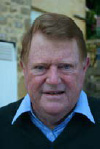Graham Pollard Employment: Emeritus Professor (Statistics), University of Canberra, Australia. Degree: PhD in Statistics. Research interests: Scoring systems in sport and in assess-ment. Optimal learning. E-mail: graham@foulsham.com.au

REFERENCES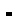Hurley W. (2007) The Ryder Cup: Are balancing four-ball pairings optimal?. Journal of Quantitative Analysis in Sports 3, 1-18.Pollard G., Pollard G., Hammond J. (2008) Proceedings of the Ninth Australasian Conference on Mathematics and Computers in Sport. Four ball best ball 2. Coolangatta, Queensland, Australia.

 Home Issues About Authors Contact Current Editorial board Authors instructions Email alerts In Press Mission For Reviewers Archive Scope Supplements Statistics Most Read Articles Most Cited Articles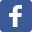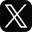This work is licensed under a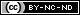Creative Commons Attribution-NonCommercial-NoDerivatives 4.0 International License.